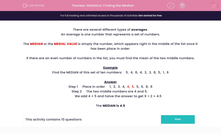# Statistics: Finding the Median

In this worksheet, students calculate the median of a given set of data.Key stage:  KS 2

Curriculum topic:   Maths and Numerical Reasoning

Curriculum subtopic:   Mean, Median, Mode and Range

Difficulty level:#### Worksheet Overview

There are several different types of averages.

An average is one number that represents a set of numbers.

The MEDIAN or the MEDIAL VALUE is simply the number, which appears right in the middle of the list once it has been place in order.

If there are an even number of numbers in the list, you must find the mean of the two middle numbers.

Example

Find the MEDIAN of this set of ten numbers:    5,  4,  8,  4,  3,  3,  8,  5,  1,  6

Step 1     Place in order     1,  3,  3,  4,  4,  5,  5,  6,  8,  8

Step 2     The two middle numbers are 4 and 5.

We add 4 + 5 and halve the answer to get 9 ÷ 2 = 4.5

The MEDIAN is 4.5

### What is EdPlace?

We're your National Curriculum aligned online education content provider helping each child succeed in English, maths and science from year 1 to GCSE. With an EdPlace account you’ll be able to track and measure progress, helping each child achieve their best. We build confidence and attainment by personalising each child’s learning at a level that suits them.

Get started